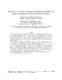## Fast, Exact, and Stable Computation of Multipole Translation and Rotation Coefficients for the 3-D Helmholtz Equation##### Files
CS-TR-4264.pdf(876.24 KB)
We develop exact expressions for translations and rotations of local and multipole fundamental solutions of the Helmholtz equation in spherical coordinates. These expressions are based on recurrence relations that we develop, and to our knowledge are presented here for the first time. The symmetry and other properties of the coefficients are also examined, and based on these efficient procedures for calculating them are presented. Our expressions are direct, and do not use the Clebsch-Gordan coefficients or the Wigner 3-j symbols, though we compare our results with methods that use these, to prove their accuracy. We test our expressions on a number of simple calculations, and show their accuracy. For evaluating a $N_t$ term truncation of the translation (involving $O(N_t^2)$ multipoles), compared to previous exact expressions that rely on the Clebsch-Gordan coefficients or the Wigner $3-j$ symbol that require $O(N_t^5)$ operations, our expressions require $O(N_t^4)$) evaluations, with a small constant multiplying the order term. The recent trend in evaluating such translations has been to use approximate "diagonalizations," that require $O(N_t^3)$ evaluations with a large coefficient for the order term. For the Helmholtz equation, these translations in addition have stabilty problems unless the accuracy of the truncation and approximate translation are balanced. We derive explicit exact expressions for achieving "diagonal" translations in $O(N_t^3)$ operations. Our expressions are based on recursive evaluations of multipole coefficients for rotations, and are accurate and stable, and have a much smaller coeffiicient for the order term, resulting practically in much fewer operations. Future use of the developed methods in computational acoustic scattering, electromagnetic scattering (radar and microwave), optics and computational biology are expected. Cross-referenced as UMIACS-TR-2001-44